Function Repository Resource:

# WignerMatrix

Get the irreducible group representation of SU(2) for a given angular momentum

Contributed by: Bernd Günther
 ResourceFunction["WignerMatrix"][j,a] computes the Wigner matrix corresponding to the matrix a representing the irreducible Lie group representation corresponding to angular momentum j.

## Details

The angular momentum j should be a non-negative integer or half-integer, while a should be a 2×2 matrix (the representation, though originally defined only on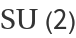, extends over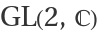).
ResourceFunction["WignerMatrix"] gives a matrix of dimension (2j+1)×(2j+1), whose entries are homogeneous polynomials of degree 2j in the entries of a.

## Examples

### Basic Examples (2)

For j=1/2, WignerMatrix yields the fundamental representation (i.e. the identity function):

 In:=Out=The matrix entries are homogeneous polynomial functions of the elements of the argument matrix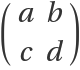:

 In:=Out=### Applications (2)

Obtain the images of the infinitesimal generators using PauliMatrix:

 In:=Out=In:=Out=In:=Out=Verify an identity:

 In:=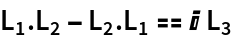Out=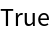### Properties and Relations (2)

For j=1 and argument matrices expressed in terms of Euler angles, the result of WignerMatrix is related to EulerMatrix through a similarity transformation:

 In:=Out=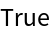For general angular momentum j, the entries of WignerMatrix are given by WignerD functions:

 In:=Out=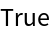### Neat Examples (4)

Check the multiplicative property of WignerMatrix:

 In:=Out=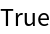The determinant of WignerMatrix with angular momentum j is the j(2j+1)th power of the determinant of the argument matrix:

 In:=Out=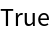The character of the representation, i.e. the trace of WignerMatrix, is given by Tr[WignerMatrix[j,x]]=Det[x]jChebyshevU[2j,Tr[x]/(2Sqrt[Det[x]])]:

 In:=Out=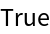As a matrix with homogeneous polynomial entries, WignerMatrix must satisfy Euler's homogeneity relation:

 In:=Out=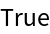bg4math

## Version History

• 1.1.0 – 28 June 2021

Unfortunately I don’t have a reference giving WignerMatrix directly in terms of the entries of the argument matrix. Costa’s and Fogli's expressions are in terms of the customary Euler angles. To derive our expression, one must resubstitute the Cayley-Klein rotation parameters from the Euler matrix in SU(2)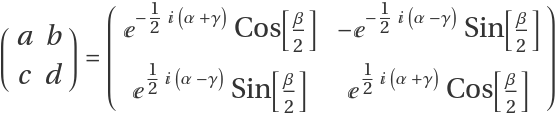, obtained from the fundamental representation j=1/2.